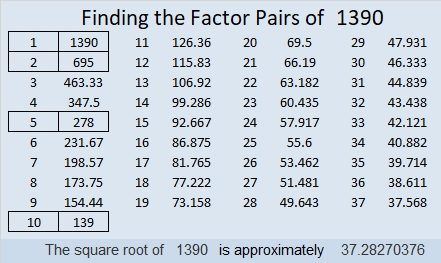# 1390 Find the Factors (ax±b)(cx±d)

I liked making a puzzle using trinomials earlier today. This one will take more skill to solve even though it contains fewer trinomials. Some of the factors will have negative numbers, and the leading coefficients of the trinomials are not 1.

In this puzzle, you can see the number 24 twice. It needs to be factored to solve the puzzle. It might be 3 × 8 or 4 × 6, but it can’t be 1 × 24 or 2 × 12 because for this puzzle ALL of the factors of 24 have to be non-zero integers from -10 to +10.Print the puzzles or type the solution in this excel file: 12 Factors 1389-1403

Every factor must appear once in the first column and once in the top row. So if you put 2x + 5 in the top row, you will also have to put 2x + 5 somewhere in the first column as well.

Sometimes all of the terms in the trinomial have a common factor and can, therefore, be factored further, but don’t worry about that right now.

You will have to find all of the factors in the puzzle before you can figure out what the missing clue should be. That’s about all the mystery I can put in a puzzle like this. Good luck with it!

Since this is different than any other puzzle I’ve ever published, you can see the solution here:Now I’ll share some information about the number 1390:

• 1390 is a composite number.
• Prime factorization: 1390 = 2 × 5 × 139
• 1390 has no exponents greater than 1 in its prime factorization, so √1390 cannot be simplified.
• The exponents in the prime factorization are 1, 1, and 1. Adding one to each exponent and multiplying we get (1 + 1)(1 + 1)(1 + 1) = 2 × 2 × 2 = 8. Therefore 1390 has exactly 8 factors.
• The factors of 1390 are outlined with their factor pair partners in the graphic below.1390 is the hypotenuse of a Pythagorean triple:
834-1112-1390 which is (3-4-5) times 278

1390 is 102345 in BASE 6 making it the smallest number to use all the digits less than 6 in base 6. Thank you OEIS.org for that reminder.